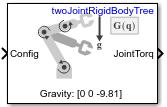# Gravity Torque

Joint torques that compensate gravity

• Library:
• Robotics System Toolbox / Manipulator Algorithms

•## Description

The Gravity Torque block returns the joint torques required to hold the robot at a given configuration with the current Gravity setting on the Rigid body tree robot model.

## Ports

### Input

expand all

Robot configuration, specified as a vector of positions for all nonfixed joints in the robot model, as set by the Rigid body tree parameter. You can also generate this vector for a complex robot using the `homeConfiguration` or `randomConfiguration` functions inside a Constant or MATLAB Function block.

### Output

expand all

Joint torques, specified as a vector. Each element corresponds to a torque applied to a specific joint. The number of joint torques is equal to the degrees of freedom (number of nonfixed joints) of the robot.

## Parameters

expand all

Robot model, specified as a `rigidBodyTree` object. You can also import a robot model from an URDF (Unified Robot Description Formation) file using `importrobot`.

The default robot model, `twoJointRigidBodyTree`, is a robot with revolute joints and two degrees of freedom.

• `Interpreted execution` — Simulate model using the MATLAB® interpreter. This option shortens startup time but has a slower simulation speed than ```Code generation```. In this mode, you can debug the source code of the block.

• `Code generation` — Simulate model using generated C code. The first time you run a simulation, Simulink® generates C code for the block. The C code is reused for subsequent simulations, as long as the model does not change. This option requires additional startup time, but the speed of the subsequent simulations is comparable to ```Interpreted execution```.

Tunable: No

## Version History

Introduced in R2018a